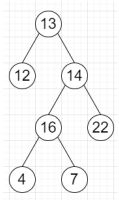ADBlock is blocking some content on the site# Program to perform an Inorder Traversal of a binary tree in Python

PythonServer Side ProgrammingProgramming

Suppose we have a binary tree; we have to find a list that contains the inorder traversal of root as a list. As we know the inorder traversal is a way of traversing all nodes in a tree where we −

• Recursively traverse the left subtree.

• Traverse the current node.

• Recursively traverse the right subtree.

We have to try to solve this problem in iterative fashion.

So, if the input is likethen the output will be [12,13,4,16,7,14,22]

To solve this, we will follow these steps −

• inorder := a new list

• stack := an empty stack

• Do the following infinitely, do

• if root is not null, then

• push root into the stack

• root := left of root

• otherwise when stack is not empty, then

• root := top element of stack and pop from stack

• insert value of root at the end of inorder

• root := right of root

• otherwise,

• come out from the loop

• return inorder

Let us see the following implementation to get better understanding −

## Example

Live Demo

```class TreeNode:
def __init__(self, value):
self.val = value
self.left = None
self.right = None
class Solution:
def solve(self, root):
inorder = []
stack = []
while True:
if root:
stack.append(root)
root = root.left
elif stack:
root = stack.pop()
inorder.append(root.val)
root = root.right
else:
break
return inorder

ob = Solution()
root = TreeNode(13)
root.left = TreeNode(12)
root.right = TreeNode(14)
root.right.left = TreeNode(16)
root.right.right = TreeNode(22)
root.right.left.left = TreeNode(4)
root.right.left.right = TreeNode(7)
print(ob.solve(root))```

## Input

```root = TreeNode(13)
root.left = TreeNode(12)
root.right = TreeNode(14)
root.right.left = TreeNode(16)
root.right.right = TreeNode(22)
root.right.left.left = TreeNode(4)
root.right.left.right = TreeNode(7)```

## Output

`[12, 13, 4, 16, 7, 14, 22]`